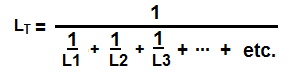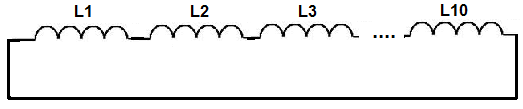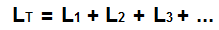﻿ Parallel and Series Inductor Calculator ﻿# Parallel and Series Inductor Calculator

### Parallel Inductor CalculatorL1: H (henries) mH (millihenries) µH (microhenries) nH (nanohenries) pH (picohenries) L2 H (henries) mH (millihenries) µH (microhenries) nH (nanohenries) pH (picohenries) L3 H (henries) mH (millihenries) µH (microhenries) nH (nanohenries) pH (picohenries) L4 H (henries) mH (millihenries) µH (microhenries) nH (nanohenries) pH (picohenries) L5 H (henries) mH (millihenries) µH (microhenries) nH (nanohenries) pH (picohenries) L6 H (henries) mH (millihenries) µH (microhenries) nH (nanohenries) pH (picohenries) L7 H (henries) mH (millihenries) µH (microhenries) nH (nanohenries) pH (picohenries) L8 H (henries) mH (millihenries) µH (microhenries) nH (nanohenries) pH (picohenries) L9 H (henries) mH (millihenries) µH (microhenries) nH (nanohenries) pH (picohenries) L10 H (henries) mH (millihenries) µH (microhenries) nH (nanohenries) pH (picohenries)

This parallel inductor calculator calculates the total parallel inductance of a circuit. This calculator allows up to 10 different inductor values. If you want to compute the total inductance of less than 10 inductors, just insert the values of the Inductors you have and leave the rest of the fields blank. For example, if you want to compute the parallel inductance of 3 inductors, just insert values into the first 3 boxes and leave the rest blank. The calculator will give you the total parallel inductance for those 3 inductors. If you want to compute the parallel inductance of more than 10 inductors, then just start with the first 10 inductors and then calculate the equivalent parallel inductance. Once you have this result, place it into one of the boxes and insert into the other boxes the remaining inductors you have in parallel. The result will be the same as if you calculated all the inductors at once.

For this calculator, a user can enter the inductance value in either unit henries (H), millihenries (mH), microhenries (μH), nanohenries (nH), or picohenries (pH). 1 millihenry is equal to 0.001 henries (H) (10-3). 1 microhenry is equal to 0.000001H (10-6). 1 nanohenry is equal to 0.000000001H (10-9). 1 picohenry is equal to 0.000000000001H (10-12).

This parallel inductor calculator calculates the total inductance, based on the formula above. The unit of the result which it gives is in unit henry (H).

For inductors in parallel, the resultant answer will always be less than the smallest inductor value, due to the nature of calculations of inductors in parallel.

### Series Inductor CalculatorL1: H (henries) mH (millihenries) µH (microhenries) nH (nanohenries) pH (picohenries) L2 H (henries) mH (millihenries) µH (microhenries) nH (nanohenries) pH (picohenries) L3 H (henries) mH (millihenries) µH (microhenries) nH (nanohenries) pH (picohenries) L4 H (henries) mH (millihenries) µH (microhenries) nH (nanohenries) pH (picohenries) L5 H (henries) mH (millihenries) µH (microhenries) nH (nanohenries) pH (picohenries) L6 H (henries) mH (millihenries) µH (microhenries) nH (nanohenries) pH (picohenries) L7 H (henries) mH (millihenries) µH (microhenries) nH (nanohenries) pH (picohenries) L8 H (henries) mH (millihenries) µH (microhenries) nH (nanohenries) pH (picohenries) L9 H (henries) mH (millihenries) µH (microhenries) nH (nanohenries) pH (picohenries) L10 H (henries) mH (millihenries) µH (microhenries) nH (nanohenries) pH (picohenries)

This series Inductor calculator calculates the total series inductance of a circuit. This calculator allows up to 10 different Inductor values. If you want to compute the total inductance of less than 10 inductors, just insert values of the inductors you have and leave the rest of the fields blank. For example, if you want to compute the series inductance of 3 inductors, just insert values into the first 3 boxes and leave the rest blank. The calculator will give you the total series inductance for those 3 inductors. If you want to compute the series inductance of more than 10 inductors, then just start with the first 10 inductors and then calculate the equivalent series inductance. Once you have this result, place it into one of the boxes and insert into the other boxes the remaining inductors you have in series. The result will be the same as if you calculated all the inductors at once.

Just like the other calculator, the user can enter the inductance values either in henries (H), millihenries (mH), microhenries (μH), nanohenries (nH), or picohenries (pH).

This series inductor calculator calculates the total inductance, based on the formula above. The unit of the result which it gives is unit henry (H).

Inductors in series simply add up. So if you have 3 inductors in series and each inductor is 1 henry, the total equivalent inductance value would be 3 henries.

Related Resources

﻿